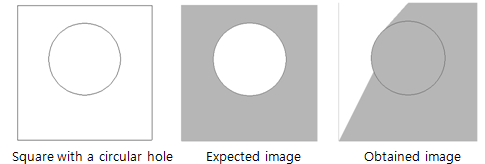# Drawing of concave polygon

Convex polygons can be rendered using

``````         glBegin(GL_POLYGON);            glEnd();.
``````

However, this does not work properly for concave polygons.
As I understand

``````          gluTessBeginPolygon(), and gluTessEndPolygon()
``````

are the functions for handling concave polygon with or without inside holes.
I’d like to know

1. Is it essential to provide user callback functions using `gluTessCallback` or is it optional?
2. If it is essential, do I have to write the callback function for every callback constant such as `GLU_TESS_BEGIN`, `GLU_TESS_BEGIN_DATA`, and so on?
3. Who are responsible for tesselation of concave polygons, OpenGL functions, or user-defined callback functions?

I am not familiar with this portion of OpenGL. So, I will appreciate it much if any one gives the answer.

I looked this up at one point. Here’s a link to some docs on the gluTess routines, and also to an alternative using the stencil buffer that lets you render concave polygons without tessellation.

It’s required. There are no defaults.

Not all are required. You need to define at least the BEGIN, VERTEX and END callbacks (or the _DATA variants). I’m not certain, but I don’t think that the COMBINE callback will be invoked unless the polygon self-intersects.

GLU deals with primitive generation. The callbacks deal with vertices. In the simplest case (no texture coordinates, colours or normals), you can just use

``````gluTessCallback(tess, GLU_TESS_BEGIN, glBegin);
gluTessCallback(tess, GLU_TESS_VERTEX, glVertex3dv);
gluTessCallback(tess, GLU_TESS_END, glEnd);
``````

If vertices have other attributes, you need to use the `GLU_TESS_VERTEX_DATA` callback instead, and call the relevant functions (glColor, glNormal, etc). The COMBINE callback (if defined) needs to interpolate all of the attributes.

Rather than re-tessellating the polygon each frame, it’s preferable to tessellate polygons once and store the resulting triangles, either in a display list or a user-defined data structure.

Thank you very much for your quick and kind answer to my question.
I followed your tips and was successful in proceeding further.
But I cannot understand what I did wrong with a simple example below.
A case of a square with an inside circular hole. The square is defined by 4 corner points, and the circle by a polygon with 100 points. (numSquarePoint=4, numCirclePoint=100)``````GLUtriangulatorObj *thePolygon;
thePolygon=gluNewTess();
gluTessCallback(thePolygon,GLU_TESS_BEGIN,(GLvoid (CALLBACK *)())glBegin);
gluTessCallback(thePolygon,GLU_TESS_VERTEX,(GLvoid (CALLBACK *)())glVertex3dv);
gluTessCallback(thePolygon,GLU_TESS_END,(GLvoid (CALLBACK *)())glEnd);
gluTessBeginPolygon(thePolygon,NULL);
gluTessBeginContour(thePolygon);
for(i=0;i<numSquarePoint;i++)
gluTessVertex(thePolygon,squareXyz[i],squareXyz[i]);
gluTessEndContour(thePolygon);
gluTessBeginContour(thePolygon);
for(i=0;i<numCirclePoint;i++)
gluTessVertex(thePolygon,circleXyz[i],circleXyz[i]);
gluTessEndContour(thePolygon);
gluTessEndPolygon(thePolygon);
``````